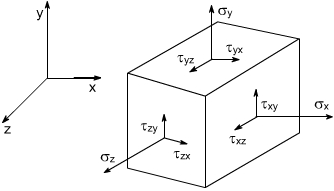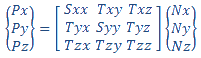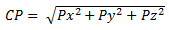Stress Components

 VON von Mises stress VONDC: von Mises [Directional Components] Available only for Linear Dynamic - Harmonic studies. The solver performs the von Mises stress calculations more accurately by taking into account the proper signs (positive or negative) of the six stress components. In most cases the VONDC: von Mises [Directional Components] stress values are expected to be higher than the von Mises stress results. P1 Normal stress in the first principal direction P2 Normal stress in the second principal direction P3 Normal stress in the third principal direction INT Stress intensity = P1 - P3 (a) with P1: maximum absolute normal stress, and P3: minimum absolute normal stress. TRI Triaxial stress = P1 + P2 + P3 (Sum of principal stress components. Also called the first stress invariant because the value does not change no matter what coordinate transformation you apply to the stress tensor.) SX Normal stress in the X-direction of the selected reference geometry SY Normal stress in the Y-direction of the selected reference geometry SZ Normal stress in the Z-direction of the selected reference geometry TXY Shear stress in the Y-direction acting on the plane normal to X-direction of the selected reference geometry For shear stress components, the first index indicates direction of surface normal, and the second index indicates direction of shear stress component. TXZ Shear stress in the Z-direction acting on the plane normal to X-direction of the selected reference geometry TYZ Shear stress in the Z-direction acting the plane normal to Y-direction of the selected reference geometry ERR Energy Norm Error CP Contact Pressure (b) ILTXZ Interlaminar shear on XZ plane ILTYZ Interlaminar shear on YZ plane(a) In some design codes and references, the Tresca equivalent stress is defined as twice the maximum shear stress which is equal to (P1 – P3), or else the stress intensity.

(b) Contact pressures are derived from the global nodal stresses by coordinate transformation. At each node, the solver reports the contact force. If the unit vector N along the direction of contact force is {Nx, Ny, Nz} in the global coordinate system, the nodal stress tensor is projected along the unit vector N to derive the three components of contact pressure {Px, Py, Pz} in the global coordinate system.The magnitude of the contact pressure CP at each node is the square root of the sum of the squares of each component. The direction of contact pressure is always normal to the area of contact.